## MP Board Class 6th Maths Solutions Chapter 4 Basic Geometrical Ideas Ex 4.3

Question 1.
Name the angles in the given figure.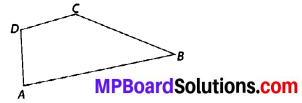Solution:
There are four angles in the given figure i.c., ∠ABC, ∠CDA, ∠DAB, ∠DCBQuestion 2.
In the given diagram, name the point(s)
(a) In the interior of ∠DOE
(b) In the exterior of ∠EOF
(c) On ∠EOF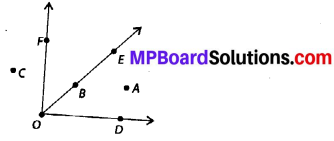Solution:
(a) Point in the interior of ∠DOE : A
(b) Points in the exterior of ∠EOF : C, A, D
(c) Points on ∠EOF : E, O, B, FQuestion 3.
Draw rough diagrams of two angles such that they have
(a) One point in common.
(b) Two points in common.
(c) Three points in common.
(d) Four points in common.
(e) One ray in common.
Solution: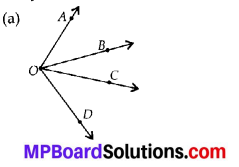Here, two angles are ∠AOD and ∠BOC and point O is common.

(b)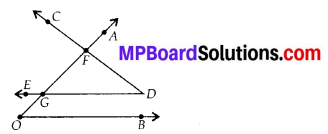Here, two angles are ∠AOB and ∠CDE and two points F and G are common.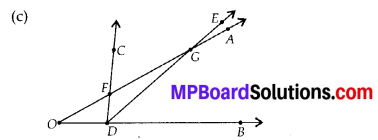Here, two angles are ∠AOB and ∠CDE and three points F, D and G are common.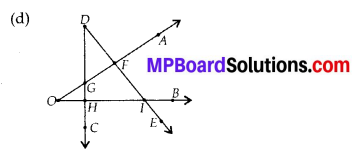Here, two angles are ∠AOB and ∠CDE and four points F, G, H and I are common.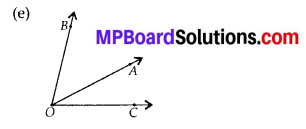Here, two angles are ∠AOB and ∠AOC and ray OA is common.